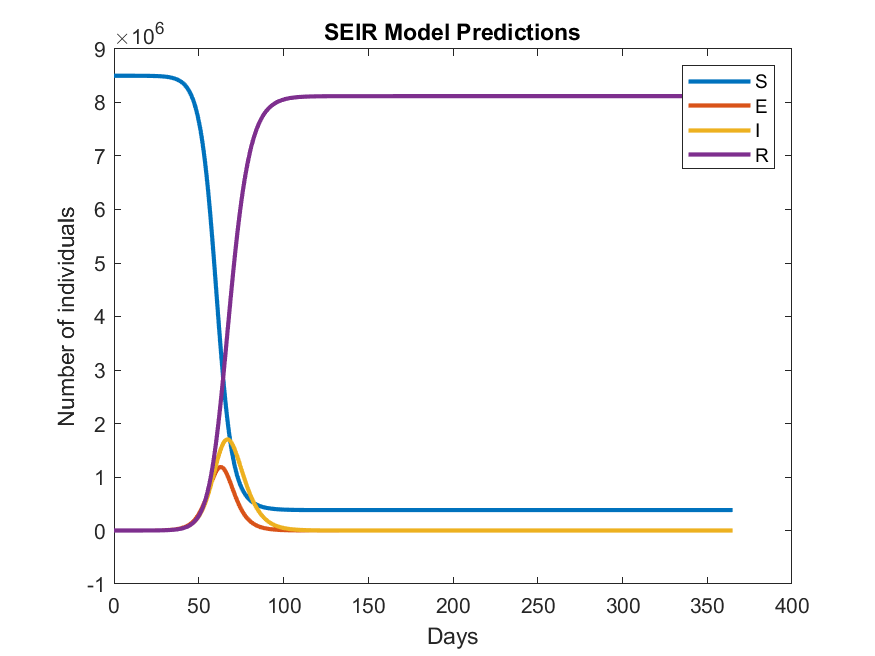## Modeling Disease Spread Using an SEIR Model

Versión 21.2.1 (85.9 KB) por
This is a simple SEIR (Susceptible-Exposed-Infectious-Recovered) model of disease spread, implemented in MATLAB®.

203 descargas

De GitHub

# Modeling Disease Spread Using an SEIR Model

This is a simple SEIR model of disease spread, implemented in MATLAB®. This approach is widely used to analyze infection data during the different stages of an epidemic outbreak. The population is divided into Susceptible (S), Exposed (E), Infectious (I), and Recovered (R) individuals.

This Live Script provides a basic SEIR model overview and an example of how you might get some graphical results using MATLAB.

The model equations in the Live Script that make up the SEIR model are based on this article.Product Requirement : MATLAB (Tested with R2021b)

This Live Script was created for the 2022 M3 Challenge Live Chat.

### Citar como

##### Compatibilidad con la versión de MATLAB
Se creó con R2021b
Compatible con cualquier versión desde R2021b